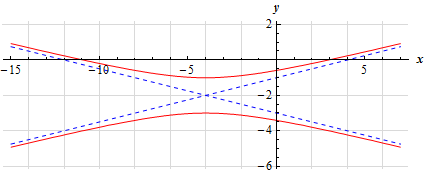Paul's Online Notes
Home / Calculus I / Review / Common Graphs
Show Mobile Notice Show All Notes Hide All Notes
Mobile Notice
You appear to be on a device with a "narrow" screen width (i.e. you are probably on a mobile phone). Due to the nature of the mathematics on this site it is best views in landscape mode. If your device is not in landscape mode many of the equations will run off the side of your device (should be able to scroll to see them) and some of the menu items will be cut off due to the narrow screen width.

### Section 1.10 : Common Graphs

18. Without using a graphing calculator sketch the graph of $$\displaystyle {\left( {y + 2} \right)^2} - \frac{{{{\left( {x + 4} \right)}^2}}}{{16}} = 1$$.

Show Solution

This is a hyperbola in standard form with the minus sign in front of the $$x$$ term and so will open up and down. The center of the hyperbola is at $$\left( { - 4, - 2} \right)$$, the two vertices are at $$\left( { - 4, - 1} \right)$$ and $$\left( { - 4, - 3} \right)$$, and the slope of the two asymptotes are $$\pm \frac{1}{4}$$.

Here is a quick sketch of the hyperbola.